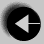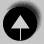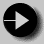## Fibonacci Series Using an Array

We have now used a variety the features of C. This final example will introduce the array. The program prints out a table of Fibonacci numbers. These are defined by a series in which any element is the sum of the previous two elements. This program stores the series in an array, and after calculating it, prints the numbers out as a table.

```
#include <stdio.h>

main()
{       int fib;
int i;

fib = 0;
fib = 1;

for(i = 2; i < 24; i++)
fib[i] = fib[i-1] + fib[i-2];

for (i = 0; i < 24; i++)
printf("%3d   %6d\n", i, fib[i]);
}```

One new construct has been introduced here.

int fib;
This defines an array called fib of 24 integers. In C, the array index is bounded by square brackets (this avoids confusion with a function call). Also, the first element of the array has index zero, and for this 24 element array, the last element is index 23.

Following this brief scan through the language, we shall introduce the components of C in rather more detail.Go to Weight conversion withGo to IndexGo to Using C with